×#### Thank you for registering.

One of our academic counsellors will contact you within 1 working day.

Click to Chat

1800-1023-196

+91-120-4616500

CART 0

• 0

MY CART (5)

Use Coupon: CART20 and get 20% off on all online Study Material

ITEM
DETAILS
MRP
DISCOUNT
FINAL PRICE
Total Price: Rs.

There are no items in this cart.
Continue Shopping• Complete JEE Main/Advanced Course and Test Series
• OFFERED PRICE: Rs. 15,900
• View Details

```Chapter 17: Combinations – Exercise 17.1

Combinations – Exercise – 17.1 – Q.1(i)Combinations – Exercise – 17.1 – Q.1(ii)Combinations – Exercise – 17.1 – Q.1(iii)Combinations – Exercise – 17.1 – Q.1(iv)Combinations – Exercise – 17.1 – Q.1(v)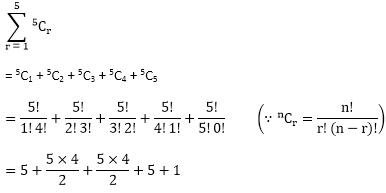= 5 + 10 +10 + 5 + 1

= 31

Combinations – Exercise – 17.1 – Q.2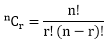Hence n = n

r = 12 and 5

Applying formula

nCp = nCq = n

Then p + q = n

⟹ nC12 = nC5

12 + 5 = n

⟹ n = 17

Combinations – Exercise – 17.1 – Q.3

If  nCp = nCq

Then p + q = n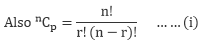⟹ nC4 = nC6

4 + 6 = n

⟹ n = 10

then 12Cn = 12C10
Applying (i)Combinations – Exercise – 17.1 – Q.4

If nCp  = nCp

The p + q = n

⟹ nC10 = nC12

10 + 12 = n

⟹ n = 22

Find 23Cn

⟹ 23C22= 23

Combinations – Exercise – 17.1 – Q.5

If nCp = nCr then P + r = n

∴ x + 2x + 3 = 24

3x = 21

x = 7

Combinations – Exercise – 17.1 – Q.6

If nCp = nCq

⟹ p + q = n

also Cx = 18Cx+2

⟹ x + x + 2 = 18

2x + 2 = 18

2x = 18 - 2 = 16

2x = 16

x = 8

Combinations – Exercise – 17.1 – Q.7

If  nCp = nCq

The p + q = n

⟹ 15C3r = 15Cr+3

4r + 3 = 15

4r = 15 - 3 = 12

r = 3

Combinations – Exercise – 17.1 – Q.8

8Cr = 7C2 + 7C3

Applying formula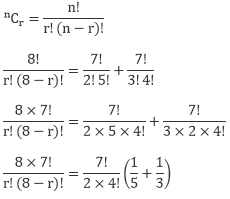Cancelling 7! from both sidesCancelling 8 on both sides

2 × 5 × 3 × 4 × 3 × 2 × 1 = r!(8 - r)!

(3 × 2)(5 × 4 × 3 × 2 × 1) = r!(8 - r)!

⟹ r! = 3!

r = 3

or

r! = 5!

r = 5

Combinations – Exercise – 17.1 – Q.9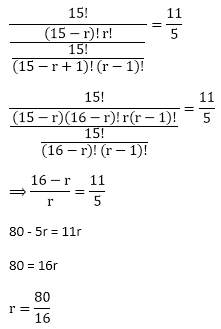80 - 5r = 11r

80 = 16r

r = 80/16

r = 5

r = 5

Combinations – Exercise – 17.1 – Q.10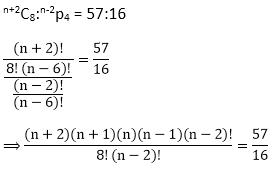Cancelling (n - 2)! from numerator and denominator

```### Course Features

• 728 Video Lectures
• Revision Notes
• Previous Year Papers
• Mind Map
• Study Planner
• NCERT Solutions
• Discussion Forum
• Test paper with Video Solution